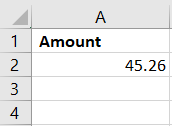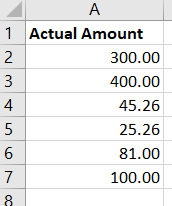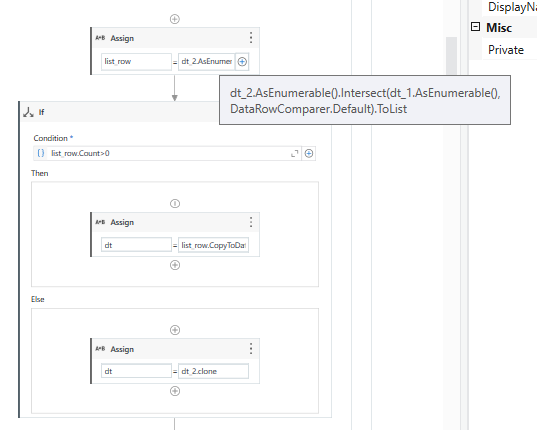# Compare column is equal or not

Hi Guys,
i need to compare a column. Input from one excel with different header and comparing data from another excel with different header. using linq i need to compare those columns any sugg!
Note: I need to look for each rows is it true or false in Comparing Data.

@Rahul_PK

Thanks

its not matches i checked already

Hi @Rahul_PK
You can compare the columns using LINQ in the following steps:

1. Load both the excel files into DataTables.
2. Use LINQ to compare the columns by creating a join between the two tables on the desired columns. For example:
``````            var query = from a in InputDataTable.AsEnumerable()
join b in ComparingDataTable.AsEnumerable()
on a.Field<string>("Amount") equals b.Field<string>("Actual Amount")
select new { a, b };
``````
1. Iterate through the result of the query and check if each row matches or not.
``````foreach (var item in query)
{
if (item.a.Field<string>("Amount") == item.b.Field<string>("Actual Amount"))
{
Console.WriteLine("True");
}
else
{
Console.WriteLine("False");
}
}

``````

Note: You may want to consider using a different data type (e.g. int, decimal, etc.) for the columns based on your data.
Thanks.

var_WorkbookB.AsEnumerable.Where(
Function(x) Not (var_WorkbookA.AsEnumerable.Select(
Function(y) y(“Code Materials”).ToString).ToArray.Contains(x(“Code Materials”).ToString)
)
).Select(Function(z) z(“Code Materials”).ToString).ToArray

i have used dt1.AsEnumerable.Where(Function(x) Double(x(“Amount”)).Equals(Double(ComparingData(“Actual Amount”))).copytodatatable i used this synatx it showing as string to interger errorthis image need to compare with the 2nd imageHi Rahul, Refer the below xaml file.
Main.xaml (11.5 KB)

Look at this thread @Rahul_PK .

Check This
Main (1).xaml (11.0 KB)

1 Like

Can u explain the code once

Refer below for explanation,
you can use the following LINQ expression to accomplish what you need:
Example screenshots:
DT1

DT2

By using the following expression, this is the output received:

Expression:
Dt2.AsEnumerable.Where(Function(x) (Dt1.AsEnumerable.Select(Function(y) y(“Amount”).ToString).ToArray.Contains(x(“Amount”).ToString))).Select(Function(z) z(“Amount”).ToString).ToArray

Where dt2 is the DT containing DT2, and DT1 is the DT containing DT1.

Hope this is what you need!

Hi, you can try thislist_row= dt_2.AsEnumerable().Intersect(dt_1.AsEnumerable(), DataRowComparer.Default).ToList

list_row is list of datarow type
dt_2 is Actual Amount, and dt_1 is Amount

Reference from this post

var result = from i in inputData.AsEnumerable()
join c in compareData.AsEnumerable()
on i[“Amount”] equals c[“Actual Amount”]
select new { IsMatch = true };